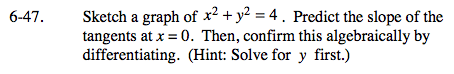### Home > CALC > Chapter 6 > Lesson 6.1.4 > Problem6-47

6-47.
1. Sketch a graph of x2 + y2 = 4 . Predict the slope of the tangents at x = 0. Then, confirm this algebraically by differentiating. (Hint: Solve for y first.) Homework Help ✎Sketch the graph (it's a circle). Then sketch the tangents (they are horizontal). What do you predict the slope of the tangents to be?

Confirm your prediction by evaluating the derivative of the circle at x = 0.